## mix BRDFs correctly

Practical and theoretical implementation discussion.
guo
Posts: 12
Joined: Thu Mar 29, 2012 4:26 am
Contact:

### mix BRDFs correctly

Hi guys,
I' m playing with nori and trying to sample BRDFs consisting of a diffuse and a glossy microfacet reflectance lobe: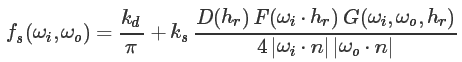where
k_d is diffuse reflectance of type Color3f
k_s equals 1 - max(k_d)
w_i is incident solid angle
w_o is outgoing solid angle
h_r is microsurface normal(half normal)
n is macrosurface normal

Implement BRDF term and PDF are straightforward:

Code: Select all


/// Evaluate the BRDF for the given pair of directions
Color3f eval(const BSDFQueryRecord &bRec) const {
if (bRec.measure != ESolidAngle
|| Frame::cosTheta(bRec.wi) <= 0
|| Frame::cosTheta(bRec.wo) <= 0)
return Color3f(0.0f);

Color3f diffuse = m_kd * INV_PI;

// Evaluate half vector
Vector3f m = h_r(bRec);

// Evaluate microfacet distribution fucntion (Beckmann)
float d = beckmann_d(m);

// Evaluate Fresnel term f
float f = fresnel(bRec.wi, m);

float g = smith_g(bRec.wi, bRec.wo, m);

Color3f glossy = m_ks * d * f * g /
(4 * std::abs(Frame::cosTheta(bRec.wi) * Frame::cosTheta(bRec.wo)));

return diffuse + glossy;
}

/// Evaluate the sampling density of sample() wrt. solid angles
float pdf(const BSDFQueryRecord &bRec) const {
if (bRec.measure != ESolidAngle
|| Frame::cosTheta(bRec.wi) <= 0
|| Frame::cosTheta(bRec.wo) <= 0)
return 0.0f;

Vector3f m = h_r(bRec);
float d = beckmann_d(m);
// po(o)
return m_kd.maxCoeff() * INV_PI * Frame::cosTheta(bRec.wo) +
m_ks * d * std::abs(Frame::cosTheta(m)) / (4.f * std::abs(m.dot(bRec.wi)));
}

At first like PBRT and Mitsuba render, monte caro weights/samples are generated as:

Code: Select all

	Color3f sample(BSDFQueryRecord &bRec, const Point2f &sample) const
{
if (Frame::cosTheta(bRec.wi) <= 0)
return Color3f(0.0f);

bRec.measure = ESolidAngle;

if (sample.y() < m_ks)
{
// Generate samples of Beckmann distribution
// Equations (28)(29) in [Wal07]
float alpha = alpha_b(bRec.wi);
float tan_theta_2_m = - alpha * alpha * std::log(sample.x()), phi_m = 2 * M_PI * sample.y() / m_ks,
cos_theta_m = 1.f / std::sqrt(tan_theta_2_m + 1), sin_theta_m = std::sqrt(tan_theta_2_m) * cos_theta_m;

// [Wal05] (5)
Vector3f m(sin_theta_m * std::cos(phi_m), sin_theta_m * std::sin(phi_m), cos_theta_m);
// Consider reflection only [Wal07] (39)
bRec.wo = 2 * std::abs(bRec.wi.dot(m)) * m - bRec.wi;
}
else
{
/* Warp a uniformly distributed sample on [0,1]^2
to a direction on a cosine-weighted hemisphere */
bRec.wo = squareToCosineHemisphere(Point2f(sample.x(), (sample.y() - m_ks) / m_kd.maxCoeff()));
}

float _pdf = this->pdf(bRec);
if (_pdf > 0.f)
{
return this->eval(bRec) * Frame::cosTheta(bRec.wo) / _pdf;
}

return Color3f(0.0f);
}

For validation, these resulting NONZERO samples are binned into 180*360 grids according to their direction, to get a empirical freqency.
And for each grid cubature method is used to integrate the above pdf() to get a corresponding ground truth frequency.
But I found deviation between these freqencies tends to larger especially when incident wi towards to grazing angle: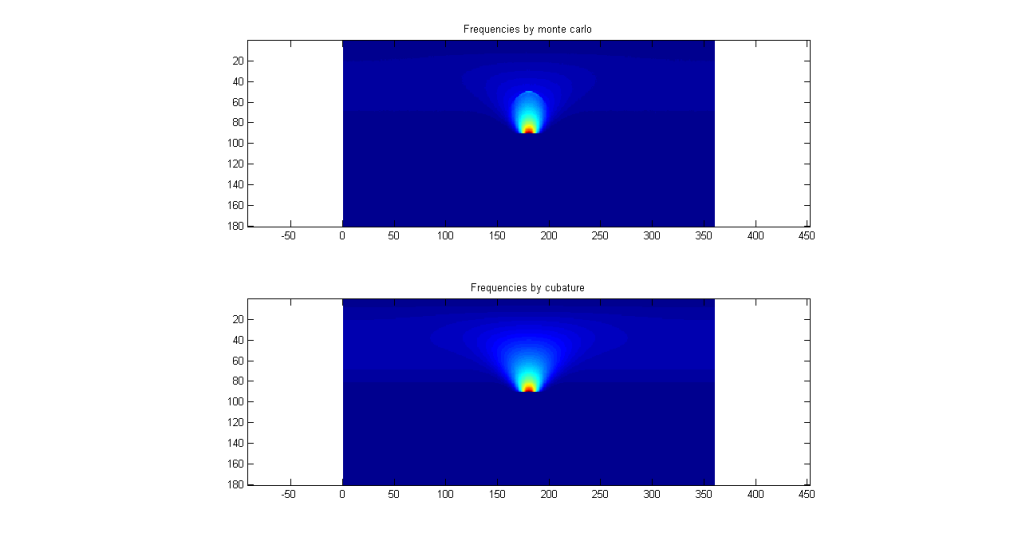I think diffuse part is not well sampled since it is dominated at this time.
So I change to the below method, which is similar to select between reflection and refraction by using Fresnel term in [Wal07]:

Code: Select all

	/// Sample the BRDF
Color3f sample(BSDFQueryRecord &bRec, const Point2f &sample) const {
if (Frame::cosTheta(bRec.wi) <= 0)
return Color3f(0.0f);

bRec.measure = ESolidAngle;

// Note m_ks + m_kd.maxCoeff() = 1.
if (sample.y() < m_ks)
{
// Generate samples of Beckmann distribution
// Equations (28)(29) in [Wal07]
float alpha = alpha_b(bRec.wi);
float tan_theta_2_m = - alpha * alpha * std::log(sample.x()), phi_m = 2 * M_PI * sample.y() / m_ks,
cos_theta_m = 1.f / std::sqrt(tan_theta_2_m + 1), sin_theta_m = std::sqrt(tan_theta_2_m) * cos_theta_m;
// [Wal05] (5)
Vector3f m(sin_theta_m * std::cos(phi_m), sin_theta_m * std::sin(phi_m), cos_theta_m);
// Equation (39) in [Wal07]
bRec.wo = 2 * std::abs(bRec.wi.dot(m)) * m - bRec.wi;

float f = fresnel(bRec.wi, m), g = smith_g(bRec.wi, bRec.wo, m);
return std::abs(bRec.wi.dot(m)) * f * g / (std::abs(Frame::cosTheta(bRec.wi) * Frame::cosTheta(m)));
}
else
{
/* Warp a uniformly distributed sample on [0,1]^2
to a direction on a cosine-weighted hemisphere */
bRec.wo = squareToCosineHemisphere(Point2f(sample.x(), (sample.y() - m_ks) / m_kd.maxCoeff()));

return m_kd;
}
}

Now the deviation is alleviated: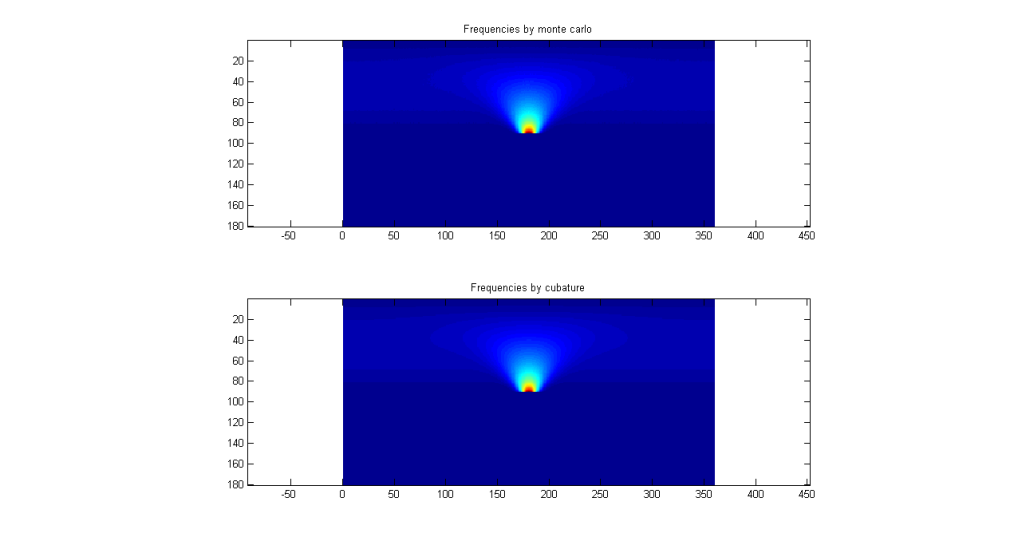It seems that the first way of mixing BRDFs is not plausible, and its underlying magic is the balance heuristic mutiple importance sampling below[Laf96], am I right?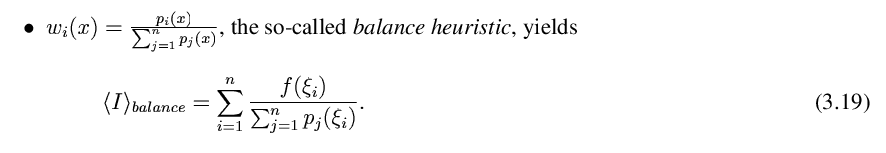Any suggestion is very appreciated.References:
[Laf96] Mathematical Models and Monte Carlo Algorithms for Physically Based Rendering
[Wal07] Microfacet Models for Refraction through Rough Surfaces

Cheers,
Guo
Last edited by guo on Thu Oct 18, 2012 1:38 am, edited 1 time in total.

guo
Posts: 12
Joined: Thu Mar 29, 2012 4:26 am
Contact:

### Re: mix BRDFs correctly

OK, let me show off a result by path racing with next event estimate: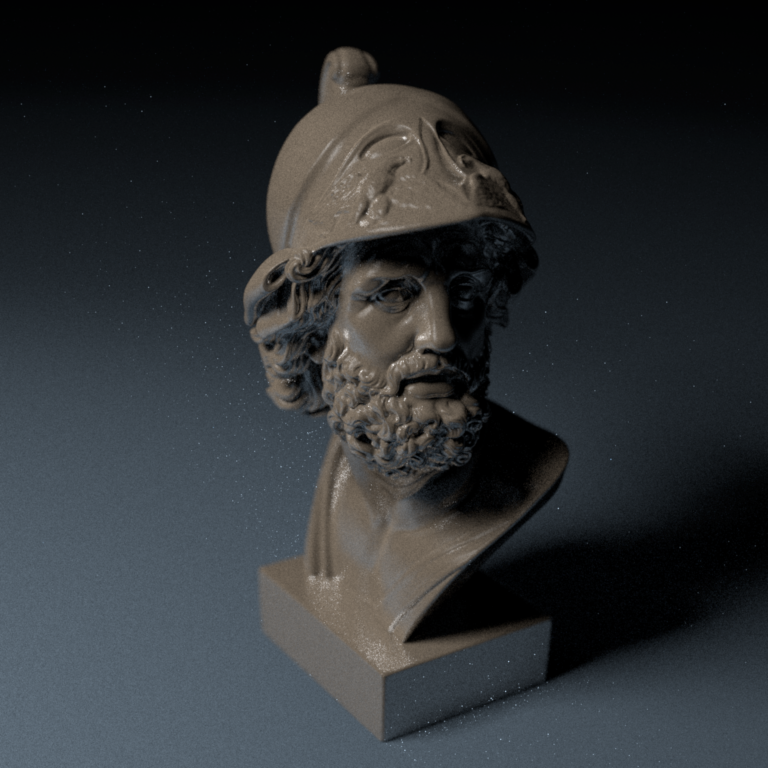256 samples are generated for each pixel.
The above eval() and pdf() are used in luminaire sampling, while sample() is used in BRDF sampling.
However, since they are different estimates, I am not sure it is reasonable this way.
Any suggestions?graphicsMan
Posts: 167
Joined: Mon Nov 28, 2011 7:28 pm

### Re: mix BRDFs correctly

I can't be 100% sure, but by visual inspection, your MIS is not being done correctly. You're simply calling pdf(bRec), but you also need to factor in the probability of the two choices. In other words, you need the two separate pdfs(bRec), and you need to combine them into a mixed pdf to normalize. You need something like eval(bRec) * cos / (m_ks*pdfBeck(bRec) + (1-m_ks)*pdfCos(bRec)).

Brian

guo
Posts: 12
Joined: Thu Mar 29, 2012 4:26 am
Contact:

### Re: mix BRDFs correctly

graphicsMan wrote:I can't be 100% sure, but by visual inspection, your MIS is not being done correctly. You're simply calling pdf(bRec), but you also need to factor in the probability of the two choices. In other words, you need the two separate pdfs(bRec), and you need to combine them into a mixed pdf to normalize. You need something like eval(bRec) * cos / (m_ks*pdfBeck(bRec) + (1-m_ks)*pdfCos(bRec)).

Brian
Hi Brian,
Thanks for your suggestion, variance seems to be lower in this way. And I think mixing samples of two estimates should be reasonable since they converge to the same expectation. But still I cannot bypass the t-test in nori render.Anyway, here is an eye candy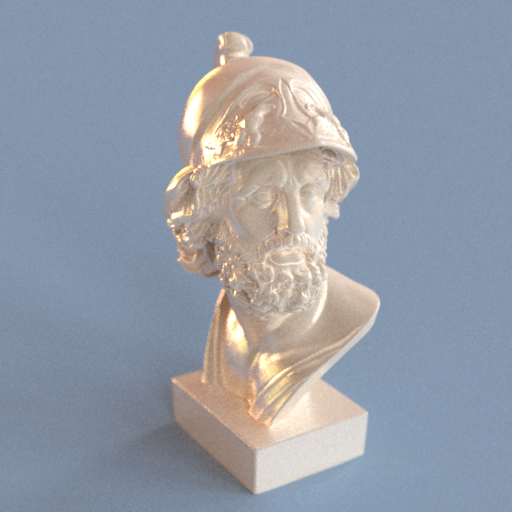Cheers,
Guo